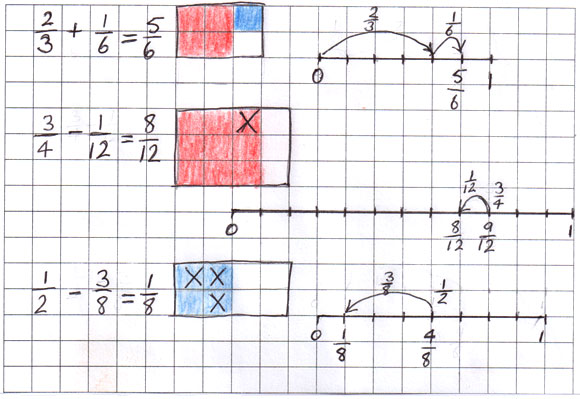Home > Fractions > Activities > Grids and jumps

# Grids and jumps

Work through at least one example with the class (e.g. $$\frac{1}{6}$$ + $$\frac{2}{3}$$) demonstrating the construction of an appropriate labelled grid diagram, a number line showing the jumps and an equation.Student work sample.

Focus on identifying the multiplicative relationship between the denominators. For example, if working with sixths and thirds, recognising that 2 $$\times$$ 3 = 6 leads to constructing a 2 $$\times$$ 3 grid to show both the sixths and the thirds.

Although using a 6 $$\times$$ 3 grid will also lead to a correct solution, encourage the students to find the smallest factor pair (making the smallest grid).

Select and prepare several sets of the task cards.

• Pairs of students select a task card and work together to present a solution.
• Grid paper can be used to support the construction of diagrams and number lines.
• Pairs who have worked on the same task cards can compare solutions and explain any differences.

As a class, discuss strategies for solving the addition or subtraction tasks without supporting diagrams.

In small groups students discuss the following scenario.

Someone in the class gave this solution: $$\frac{3}{10}$$ – $$\frac{1}{5}$$ = $$\frac{2}{5}$$.

How would you explain why the answer is incorrect? Share some explanations as a class.